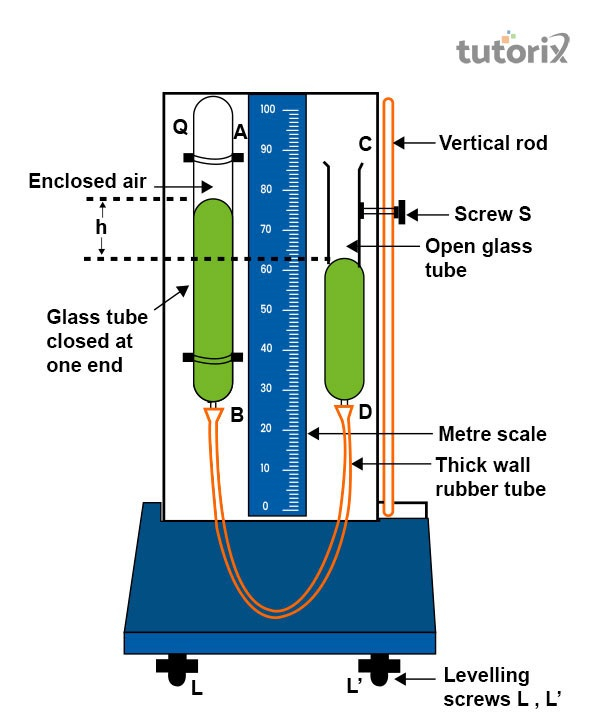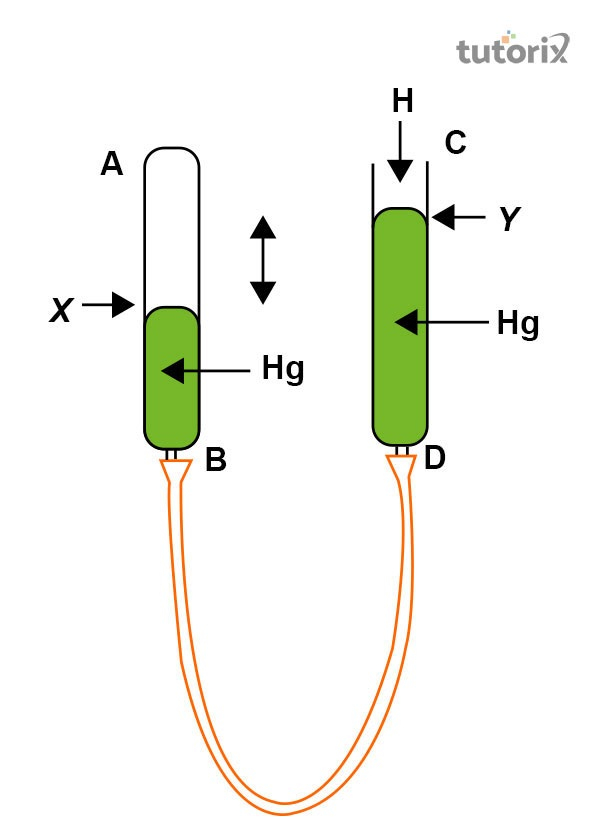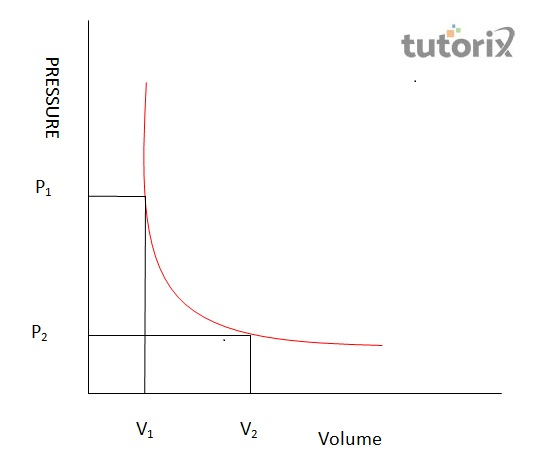# To study the variation in volume with pressure for a sample of air at constant temperature by plotting graphs between p and v

## Introduction

Theory of Boyle’s law is based on the properties of several gases and their laws. For any gaseous substances, it is stated that the gaseous matter does not have any proper shape or volume and it seems to adopt the shape where it is contained. The primary macroscopic properties of gases include their volume, pressure, mass and temperature respectively. All these properties of gasses can be easily explained by the theory of kinetic theory, where the respective motions and molecular composition of gases are considered.

## Theory of Boyle’s law

Prior to defining of Theory of Boyle’s law, the laws of gases are to be explained. The Law of gas is defined to show the intricate relationship that existed between the macroscopic properties of gases (Lin et al. 2021). However, Theory of Boyle’s law states the increase that is noticed within the volume of the given gaseous matter with a decrease in the pressure for the stated, gaseous matter.Figure 1: Boyle’s law apparatus

This law further states that the pressure of the given mass of gas is considered to be inversely proportional to the volume of the gaseous matter. This condition is stated to be observed when the temperature for the given mass of gas is kept constant.

## Aims and apparatus required

The aim of this practical experiment states that the change in the pressure and volume, the macroscopic properties that are noted for the given sample of air. However, this experiment should be conducted by keeping the temperature constant for the stated air sample (Amrita.olabs.edu, (2022). Furthermore, a graph will be plotted between changes in pressure and volume that are identified in this practical experiment. In this experiment, the apparatus required includes the apparatus of Boyle’s law, plumb line, Fortin's barometer, a pair for set squares and lastly a thermometer.

## Lab Procedures

Certain lab procedures are to be maintained which are as follows. Firstly, the apparatus is to be set vertically and is supported with a heavy metallic base and screws for levelling. Plumb lines are used for testing the apparatus used. Tube A consisted of the enclosed air, and mercury lies in the tube B and atmospheric pressure is to be noted in the Fortin’s barometer with the temperature.Figure 2: Pressure of air in tube AB = H + h

The volume will be noted for tube A which is graduated. However, both tubes A and B are moved for noting down the variations that are noticed in the pressure and volume for the air sample.

## Observations

Position of Hg levelPressure difference (p) (cm)Tube-A and Tube-B Pressure of air P = P0+p (cm of Hg)Volume of air V (cm3)1/V (cm-1)PV
In Tube-A (cm)In Tube-b (cm)

Table 1: Studying the variation in volume with pressure

## Result

According to the observations from studying the variation with pressure, the PV stands constant. This mostly takes place due to the application of Boyle’s law in the process. Here, the P-V graph appears as a hyperbola and eventually helps in satisfying the equal relation between the PV and the constant pressure.Figure 3: Boyle’s law

This also satisfies the relation $\mathrm{P \varpropto 1/V}$ that helps in studying the variation of the volume with pressure.

## Precautionary measures

The study regarding the variation in the volume needs to follow a few precautions that can be successfully conducted the study. First, the plotting of the graph needs to be done with utmost authenticity. After that, it should be noted that the wooden board is vertical and the mercury and set-squares are taken properly during the test (Kim, Kim & Han, 2021). Immediately after that, the atmospheric pressure needs to be calculated from the beginning to the end of the experiment.

## Conclusion

The study regarding the volume with the pressure of air incorporates Boyle’s law where the volume and the pressure are inversely proportional to each other. In the study process, the tube-A needs to be kept pure and dry in the fresh air for maintaining utmost authenticity. The result can be concluded on the part where the P-1 is divided by a V graph with a straight line that has a positive slope at the end.

## FAQs

Q1. How does the changing volume affect pressure when the temperature is constant?

Ans. The changing volume and pressure have a proportional relationship where the gradual decrease of contained gas can increase the pressure and the increase of volume will decrease the pressure. The process appears vice versa for both factors while studying the variation between those factors.

Q2. How does the volume of gas change when pressure is applied to it?

Ans. The volume of gas changes proportionally when certain pressure is applied to it. After pressure is applied to gas, it increases immediately and consequently the volume of the gas decreases.

Q3. Does pressure have any effect on the increasing and decreasing of volume?

Ans. The particles of gas start colliding on the walls of the container when the volume of the gas is decreased. In this scenario, the particles on the walls start exerting a force which eventually controls the increasing and decreasing of volume.

Q4. What are some of the possible sources for errors during the verification in volume with pressure?

Ans. The verification process comes with significant sources of errors like the usage of impure air in the Tube-A. In addition, the narrow base and the less stability of the apparatus can be major sources of error.